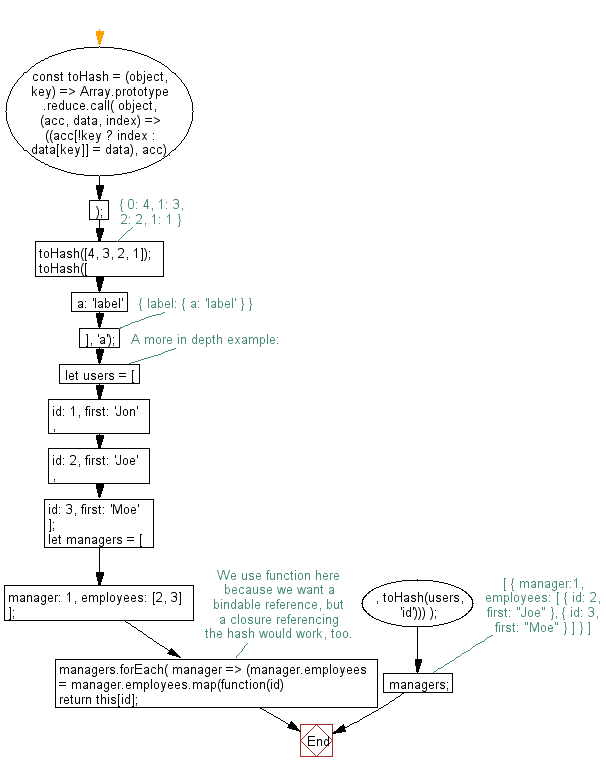# JavaScript: Reduce a given Array-like into a value hash

## JavaScript fundamental (ES6 Syntax): Exercise-124 with Solution

Write a JavaScript program to reduce a given Array-like into a value hash (keyed data store).

Note: Given an Iterable or Array-like structure, call Array.prototype.reduce.call() on the provided object to step over it and return an Object, keyed by the reference value.

• Given an iterable object or array-like structure, call Array.prototype.reduce.call() on the provided object to step over it and return an Object, keyed by the reference value.

Sample Solution:

JavaScript Code:

``````//#Source https://bit.ly/2neWfJ2
const toHash = (object, key) =>
Array.prototype.reduce.call(
object,
(acc, data, index) => ((acc[!key ? index : data[key]] = data), acc),
{}
);
toHash([4, 3, 2, 1]); // { 0: 4, 1: 3, 2: 2, 1: 1 }
toHash([{ a: 'label' }], 'a'); // { label: { a: 'label' } }
// A more in depth example:
let users = [{ id: 1, first: 'Jon' }, { id: 2, first: 'Joe' }, { id: 3, first: 'Moe' }];
let managers = [{ manager: 1, employees: [2, 3] }];
// We use function here because we want a bindable reference, but a closure referencing the hash would work, too.
managers.forEach(
manager =>
(manager.employees = manager.employees.map(function(id) {
return this[id];
}, toHash(users, 'id')))
);
managers; // [ { manager:1, employees: [ { id: 2, first: "Joe" }, { id: 3, first: "Moe" } ] } ]
console.log(managers);
```
```

Sample Output:

```[{"manager":1,"employees":[{"id":2,"first":"Joe"},{"id":3,"first":"Moe"}]}]
```

Flowchart:Live Demo:

See the Pen javascript-basic-exercise-124-1 by w3resource (@w3resource) on CodePen.

Improve this sample solution and post your code through Disqus

What is the difficulty level of this exercise?

Test your Programming skills with w3resource's quiz.

﻿

## JavaScript: Tips of the Day

Chunks an array into n smaller arrays

Example:

```const tips_chunkIntoN = (arr, n) => {
const size = Math.ceil(arr.length / n);
return Array.from({ length: n }, (v, i) =>
arr.slice(i * size, i * size + size)
);
}
console.log(tips_chunkIntoN([1, 2, 3, 4, 5, 6, 7,8], 4));
```

Output:

```[[1,2],[3,4],[5,6],[7,8]]
```